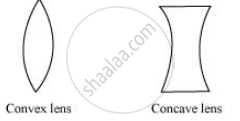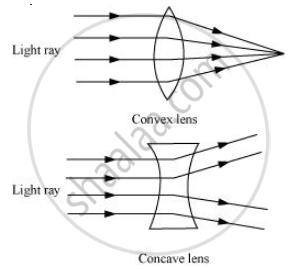Share

# State Two Differences Between a Convex and a Concave Lens. - CBSE Class 7 - Science

ConceptImages Formed by Lenses

#### Question

State two differences between a convex and a concave lens.

#### Solution 1

1) Convex lens is thicker in the middle while concave lens is thinner in the middle.2) A convex lens converges the light ray falling on it, while a concave lens diverges the light ray falling on it.#### Solution 2

 Convex lens Concave lens Convex lens can form both real and virtual images Concave lens always forms a virtual image It can form magnified image. Image is always diminished in size.
Is there an error in this question or solution?

#### APPEARS IN

Solution State Two Differences Between a Convex and a Concave Lens. Concept: Images Formed by Lenses.
S